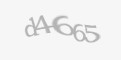## test latex

MathJax TeX Test PageWhen $a \ne 0$, there are two solutions to $$ax^2 + bx + c = 0$$ and they are $$x = {-b \pm \sqrt{b^2-4ac} \over 2a}.$$